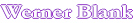Home     Calculate the Viscosity of a Polymer Melt or Solution over a Temperature Range For the polymer chemist the glass transition temperature (Tg) is a useful property. The glass transition temperature of the polymer or oligomer can be related to the viscosity of the polymer solution and the viscosity of the polymer at different temperatures. The Tg of a polymer can be understood in terms of the free volume concept. A polymer at Tg or below its Tg has no free volume for the polymer chains to move. Heating a polymer above its Tg increases the free volume and makes the mobility of the polymer chains possible. This concept of the free volume is expressed in the WLF[i] equation (William, Landel and Ferry). Below is a modification of this equation, which uses universal constants according to Nielsen[ii]. These universal constants are reasonable accurate for most polymers. If a deviation is found for a series of polymers these constants can be readjusted. Measured Data WLF equation Log η= C-A(T-Tg)/(B+(T-Tg)) °C VISC cps POISE VISC LOG T-TG Tg °C C= Universal Constants for most polymers Polymer A A= Polymer B B= Enter desired range Delta, temp Calculated Value Temp.°C Viscosity, cps Comp A Comp B Log (poise) Comp A Comp B [i] M.L.Williams, R.F.Landel and  J.D.Ferry, J.Am.Chem.Soc.77,3701(1955) [ii] L.E.Nielsen, Polymer Rheology, Marcel Dekker, 1977,pp.33,74,133

Automatic recalculation

 Last edited on: October 21, 2010 Copyright®, Design, Layout and Technical Content by: